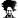# Userform.Textbox: Get the cursor position's entire word

#### inddon

##### Member
Hello There,

I have a userform with the Textbox. I would like to know the VBA code to get the entire word where the cursor is placed.

I have attached a sample workbook for your reference.

Thank you & regards,
Don

#### Attachments

• 13.4 KB Views: 15

#### Hui

##### Excel Ninja
Staff member

Code:
``````Private Sub CommandButton1_Click()

Dim MyStr As String
MyStr = Replace(TextBoxComments.Text, Chr(10), " ")
MyStr = Replace(MyStr, Chr(13), " ")

Dim last_spc As Integer, first_spc As Integer

last_spc = InStr(TextBoxComments.SelStart, MyStr, " ")
lftpiece = Left(MyStr, last_spc)
first_spc = InStrRev(lftpiece, " ", Len(lftpiece) - 1) + 1

a = MsgBox(Mid(lftpiece, first_spc, Len(lftpiece) - first_spc), vbOKOnly)

End Sub``````
enjoy

•inddon

#### inddon

##### Member

Code:
``````Private Sub CommandButton1_Click()

Dim MyStr As String
MyStr = Replace(TextBoxComments.Text, Chr(10), " ")
MyStr = Replace(MyStr, Chr(13), " ")

Dim last_spc As Integer, first_spc As Integer

last_spc = InStr(TextBoxComments.SelStart, MyStr, " ")
lftpiece = Left(MyStr, last_spc)
first_spc = InStrRev(lftpiece, " ", Len(lftpiece) - 1) + 1

a = MsgBox(Mid(lftpiece, first_spc, Len(lftpiece) - first_spc), vbOKOnly)

End Sub``````
enjoy
Hi Hui,

Thank you for taking the time for providing the code. Please refer the attached snapshot for cases it is giving issues.Look forward to hearing from you.

Regards,
Don

#### Hui

##### Excel Ninja
Staff member
I never liked the original code but I was in a hurry
Try the following:

Code:
``````Private Sub CommandButton1_Click()
Dim ans As String
Dim MyStr As String

MyStr = Replace(TextBoxComments.Text, Chr(10), " ")
MyStr = Replace(MyStr, Chr(13), "")

Dim s As Variant
s = Split(MyStr, " ")

Dim comstr As String
comstr = ""

Dim i As Integer
For i = 0 To UBound(s)
comstr = comstr + s(i)

ans = s(i)
Exit For
End If

comstr = comstr + " "
Next i

a = MsgBox(ans)

End Sub``````

•inddon

#### inddon

##### Member
I never liked the original code but I was in a hurry
Try the following:

Code:
``````Private Sub CommandButton1_Click()
Dim ans As String
Dim MyStr As String

MyStr = Replace(TextBoxComments.Text, Chr(10), " ")
MyStr = Replace(MyStr, Chr(13), "")

Dim s As Variant
s = Split(MyStr, " ")

Dim comstr As String
comstr = ""

Dim i As Integer
For i = 0 To UBound(s)
comstr = comstr + s(i)

ans = s(i)
Exit For
End If

comstr = comstr + " "
Next i

a = MsgBox(ans)

End Sub``````

Thank you Hui for taking the time in providing the code. It works wonderful!Regards,
Don